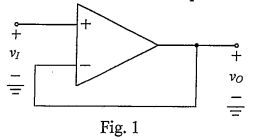lvy>試卷(2022/08/12)

# 105 年 - 105 國立中山大學_碩士班招生考試_電機系(甲組)(己組)：電子學#110214

【非選題】
1.

1. (10%) An operational amplifier has a rated output voltage of ±10 V and a slew rate (SR) of 2 V/μs.【題組】(a) What is its full-power bandwidthfM?

【非選題】
2.【題組】 (b) If an input sinusoidal signal with frequency f = 5fM is applied to a unity-gain follower configuration as shown in Fig. 1, what is the maximum possible amplitude that can be accommodated at the output without incurring SR distortion? (5%*2)

【非選題】
3.

2.(10%) Consider a circuit of Fig. 2 with a BJT device having β = 100 when it is biased in the active region. The constant voltage drop 0.7 V approximation can be used for the turn-on of a p-n junction, and the constant voltage drop~ 0.2 V approximation can be used when the BJT is biased in the saturation region. Please calculate the collector current Ic and base current IB. (5%*2)【非選題】
4.

3. (15%) Consider the voltage amplifier of Fig. 3(a) with Voo = 2 V, Rp = 20 kQ, and with a MOSFET specified to have Vt = 0.5 V,(W/L) = 5 mA/V2, and λ = 0. Calculate theof the voltage transfer characteristic as shown in Fig. 3(b). (5%*3)【非選題】
5.

4. (15%) Consider the yoltage amplifier of Fig. 4 with a MOSFET specified to have Vt=  1.5 V,(WIL) = 0.5 mArV2, andλ = 0.02. The coupling capacitors C can block DC component and pass AC component. Calculate (a) the small-signal voltage gain(b) its input resistance, and (O) the largest allowable input signal. (5%*3)【非選題】
6.

5. (25%) Consider the voltage amplifier of Fig. 5 with two BJT devices: Q1 and Q2, having β= 100 when they are biased in the active region. The coupling capacitors C can block DC component and pass AC component. The voltage supplies are 10 V and the thermal voltage VT = 25.9 mV. The constant voltage drop 0.7 V approximation can be used for the turn-on of a p-n junction. In addition, other resistances are also included in the Fig. 5. Please come out the following parameters: (a) DC base voltage of Q1:, (b) DC base voltage of Q2:, (c) DC collector voltage of Q2:. (d) overall AC voltage gain. (5%, 5%, 5%, 10%)【非選題】
7.

6. (25%) Consider the voltage amplifier of Fig. 6 with a BJT device having β = 100 when it is biased in the active region. The coupling capacitors C1 = C2 = C3 = 1 μF. The voltage supplies are 5 V and the thermal voltage VT= 25.9 mV. If the transistor is specified to have fT = 700 MHz and Cμ = 1 pi. The constant voltage drop 0.7 V approximation can be used for the turn-on of a p-n junction. In addition. other resistances are also included in the Fig. 6. Please calculate (a)f1，f2，f3 (let f1 ＜f2＜f3) values of the low-frequcncy transfer function(b) the upper 3-dB frequcncy fHby using open-circuit time constants method if the ro and rx effect are neglected. (5%*3, 10%)### 懸賞詳解

#### 國二理化上第三次

19、下列何者並不是「道耳頓原子說」的內容？ (A)原子為最小粒子，不可再分割 (B)化學變化只是原子重新排列組 合 (C)不同元素的原子能以簡單整數比�...